Practical Geometry
Serial order wise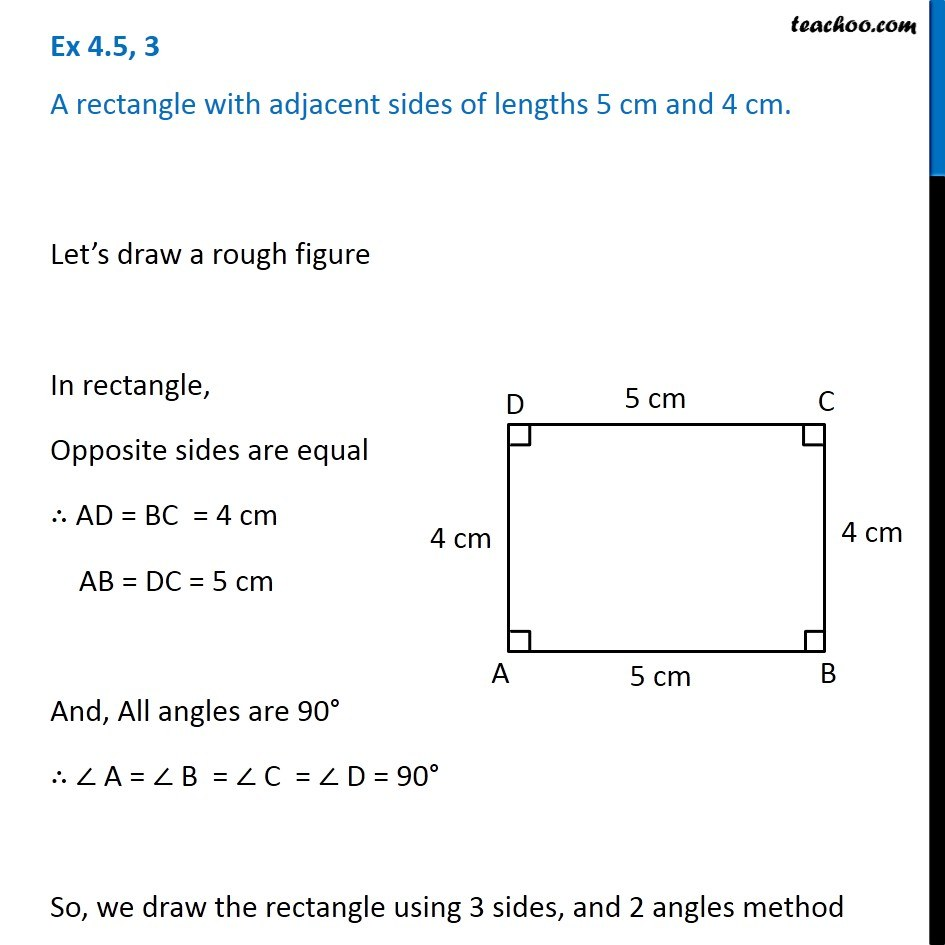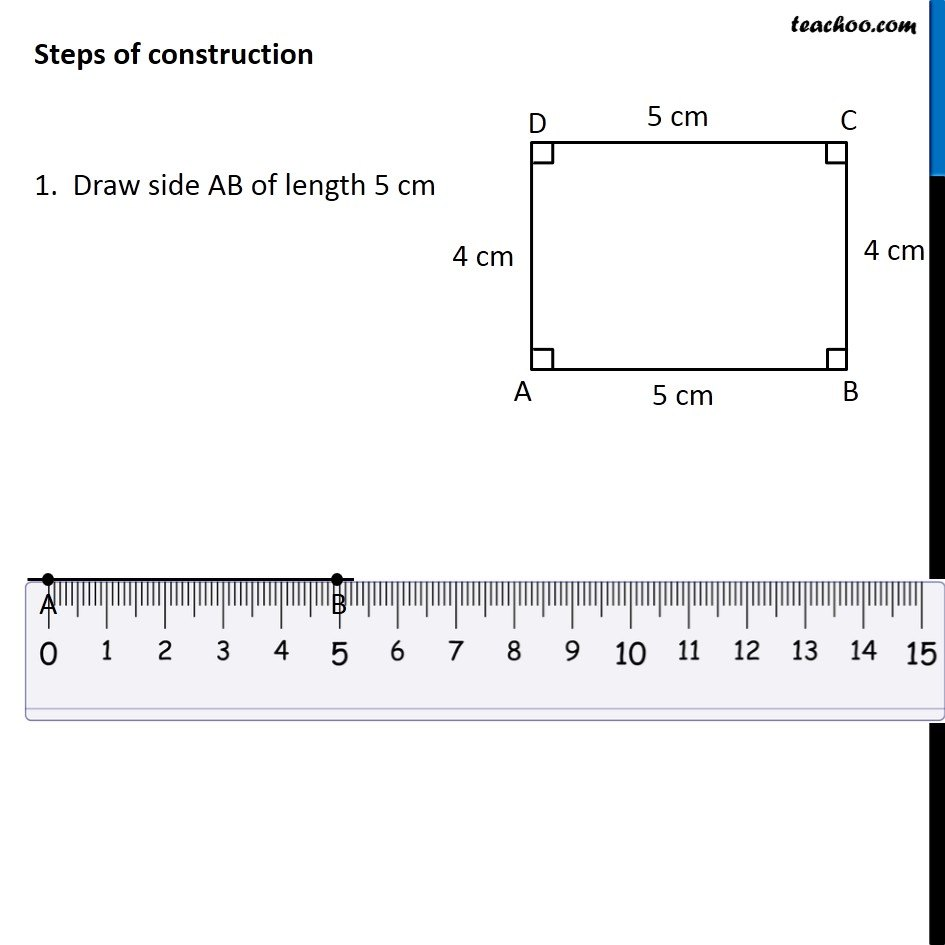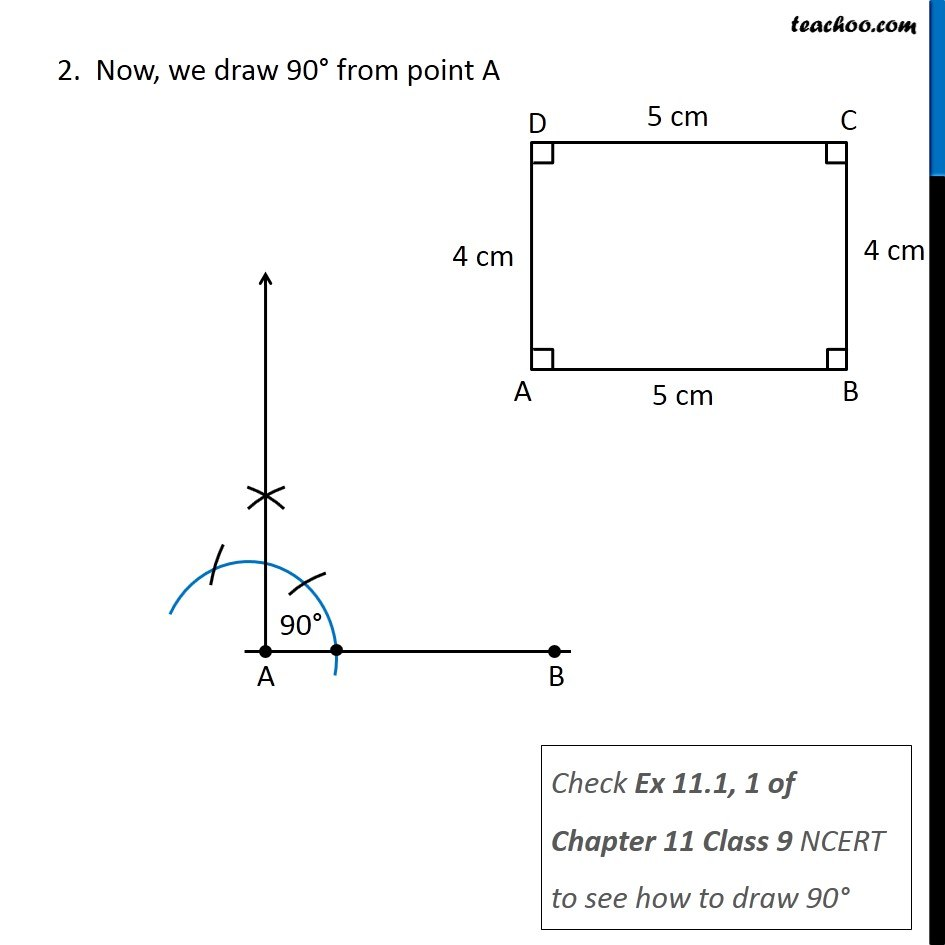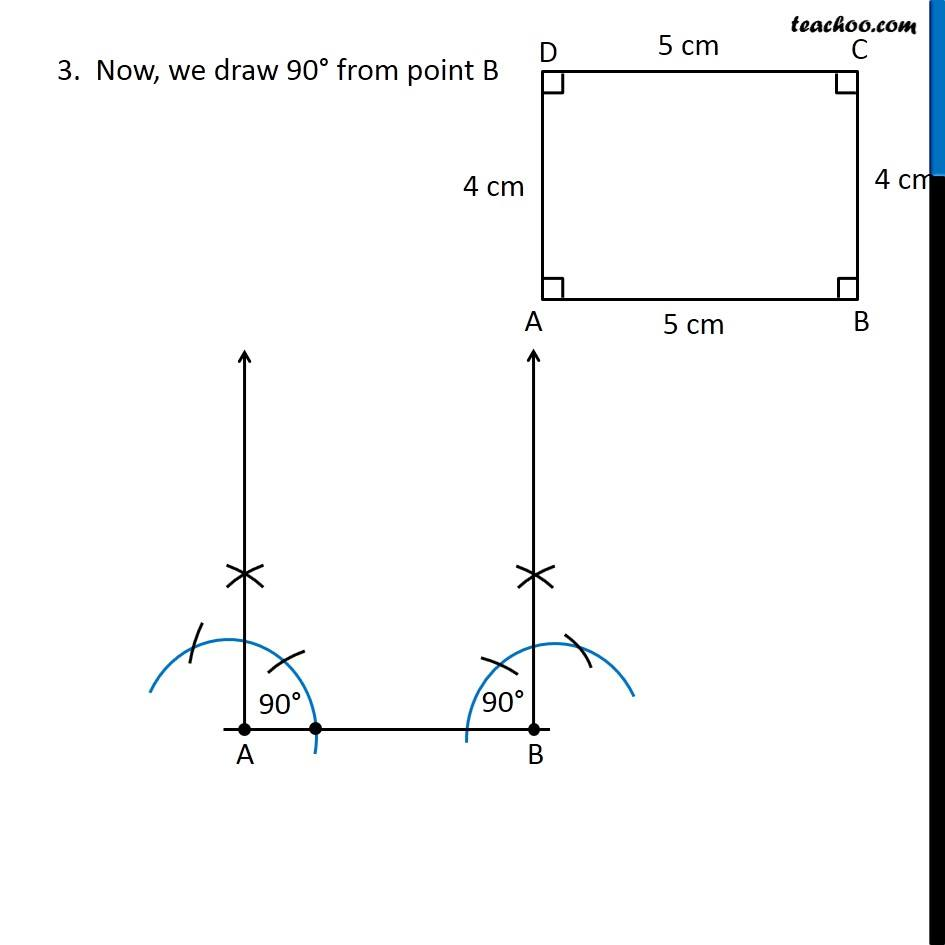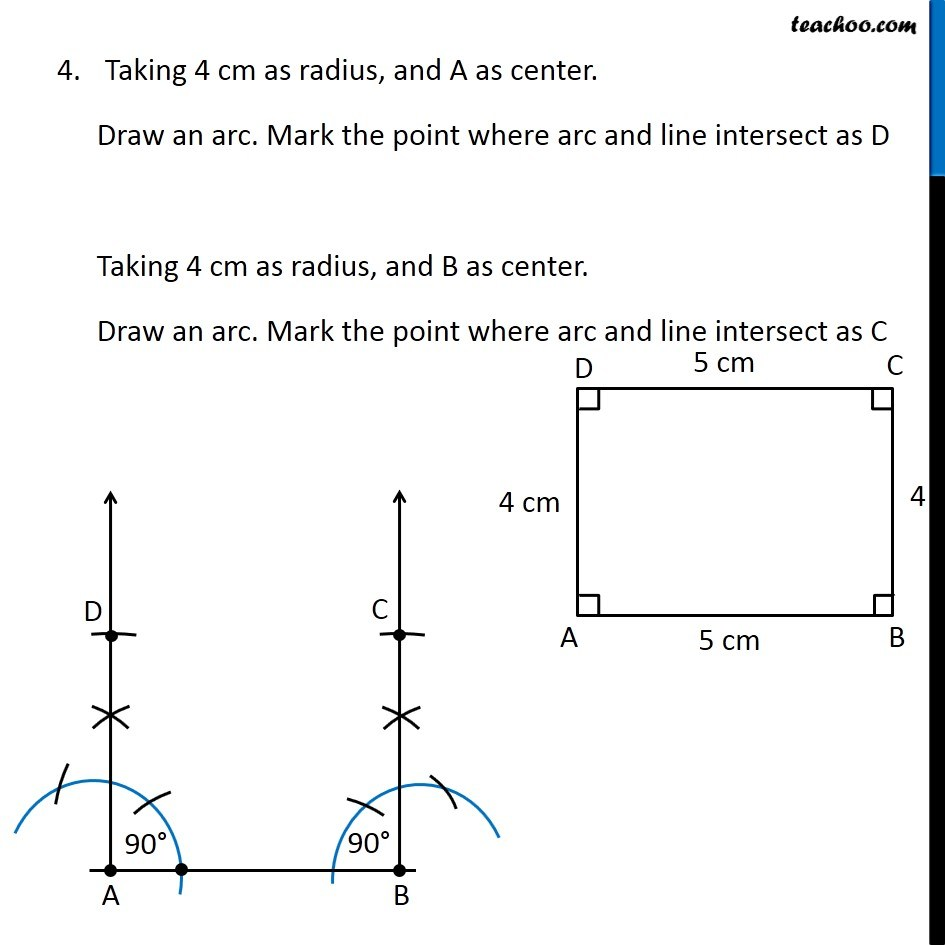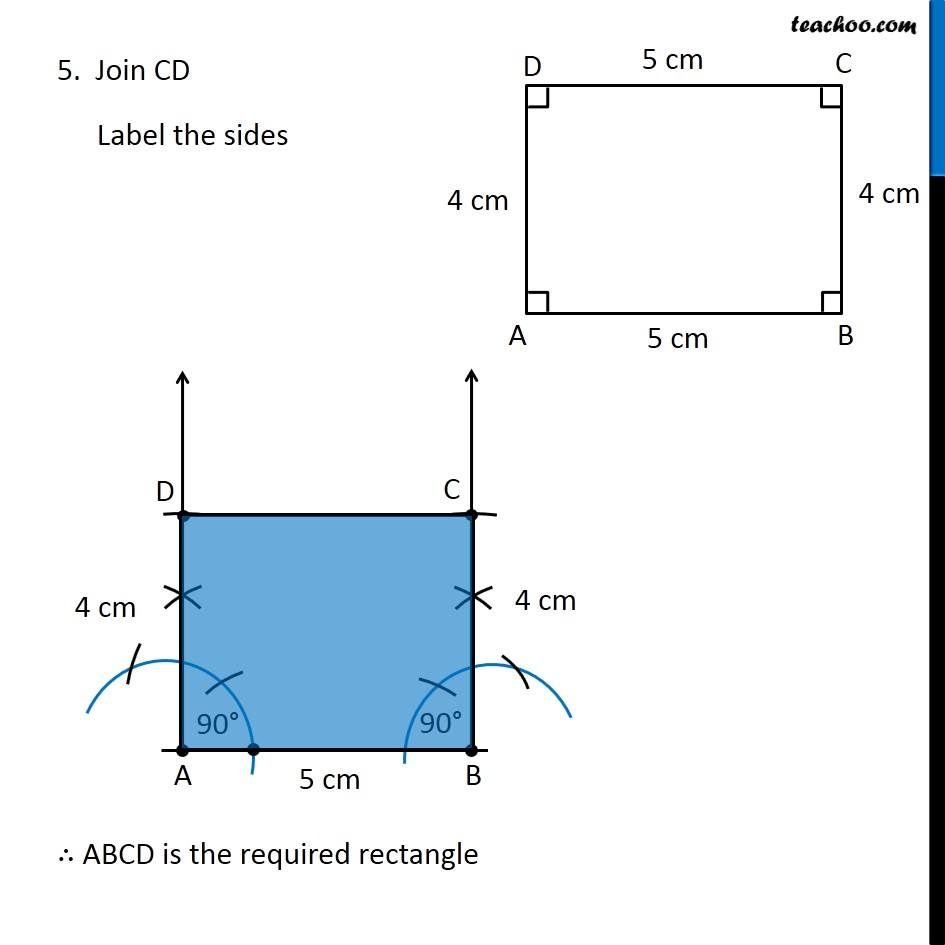Learn in your speed, with individual attention - Teachoo Maths 1-on-1 Class

### Transcript

Question 3 A rectangle with adjacent sides of lengths 5 cm and 4 cm. Let’s draw a rough figure In rectangle, Opposite sides are equal ∴ AD = BC = 4 cm AB = DC = 5 cm And, All angles are 90° ∴ ∠ A = ∠ B = ∠ C = ∠ D = 90° So, we draw the rectangle using 3 sides, and 2 angles method Steps of construction 1. Draw side AB of length 5 cm 2. Now, we draw 90° from point A Check Ex 11.1, 1 of Chapter 11 Class 9 NCERT to see how to draw 90° 3. Now, we draw 90° from point B 4. Taking 4 cm as radius, and A as center. Draw an arc. Mark the point where arc and line intersect as D Taking 4 cm as radius, and B as center. Draw an arc. Mark the point where arc and line intersect as C 5. Join CD Label the sides ∴ ABCD is the required rectangle